# De Rham theorem

A theorem expressing the real cohomology groups of a differentiable manifoldin terms of the complex of differential forms (cf. Differential form) on. If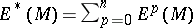is the de Rham complex of, whereis the space of all infinitely-differentiable-forms onequipped with the exterior differentiation, then de Rham's theorem establishes an isomorphism between the graded cohomology algebraof the complexand the cohomology algebra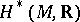ofwith values in. An explicit interpretation of this isomorphism is that to each closed-formthere is associated a linear form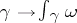on the space of-dimensional singular cyclesin.
There are various versions of de Rham's theorem. For example, the cohomology algebraof the complex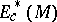of forms with compact supports is isomorphic to the real cohomology algebra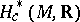of the manifoldwith compact supports. The cohomology ofwith values in a locally constant sheaf of vector spaces is isomorphic to the cohomology of the complex of differential forms with values in the corresponding flat vector bundle . The cohomology of a simplicial setwith values in any fieldof characteristic 0 is isomorphic to the cohomology of the corresponding de Rham polynomial complex over. In the case whenis the singular complex of an arbitrary topological spaceone obtains in this way a graded-commutative differential graded-algebra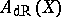with cohomology algebraisomorphic to the singular cohomology algebra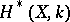(see ). Ifis a smooth affine algebraic variety over, then the cohomology algebra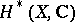is isomorphic to the cohomology algebra of the complex of regular differential forms on(see de Rham cohomology).